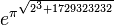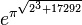# Difference between revisions of "Sandbox"$e^{\pi^{\sqrt{2^3 + 1729323232}}}$$e^\pi + 21$$3$$e^{\pi^{\sqrt{2^3 + 17292}}}$